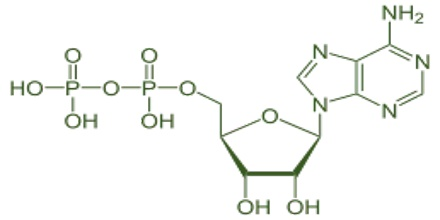Oxyanion

Definition

Oxyanion (or oxoanion) is a chemical compound with the generic formula AxOyz−, where A represents a chemical element and O represents an oxygen atom. Oxoanions are formed by a large majority of the chemical elements. The formulae of simple oxoanions are determined by the octet rule. The structures of condensed oxyanions can be rationalized in terms of AOn polyhedral units with sharing of corners or edges between polyhedra. The phosphate and polyphosphate esters AMP, ADP and ATP are important in biology.Here, Nitrate (NO3), Nitrite (NO2), sulfite (SO32-) and hypochlorite (ClO) are all oxyanions.

Some elements are able to form more than one oxyanion, polyatomic ions that contain oxygen, each containing a different number of oxygen atoms. For example, chlorine can combine with oxygen in four ways to form four different oxyanions: ClO4, ClO3, ClO2, and ClO.

Formula and Structure

The formula of monomeric oxoanions, AOm−n, is dictated by the oxidation state of the element A and its position in the periodic table. Elements of the first row are limited to a maximum coordination number of 4. However, none of the first row elements has a monomeric oxoanion with that coordination number. Instead, carbonate (CO2−3) and nitrate (NO3) have a trigonal planar structure with π bonding between the central atom and the oxygen atoms. This π bonding is favoured by the similarity in size of the central atom and oxygen. Many oxoanions of elements in lower oxidation state obey the octet rule and this can be used to rationalize the formulae adopted.The most common of the chlorine oxyanions is chlorate, ClO3.  Chlorine can combine with oxygen in four ways to form four different oxyanions: ClO4-, ClO3-, ClO2-, and ClO. The formula of the oxoanion, SiO2−3 is obtained as follows: each nominal silicon ion (Si4+) is attached to two nominal oxide ions (O2−) and has a half share in two others. Thus the stoichiometry and charge are given by:

Stoichiometry: Si + 2 O + (2 ×  12) O = SiO3

Charge: +4 + (2 × −2) + (2 × ( 12 × −2)) = −2.

A polyoxoanion is a polymeric oxoanion in which multiple oxoanion monomers, usually regarded as MOn polyhedra, are joined by sharing corners or edges. When two corners of a polyhedron are shared the resulting structure may be a chain or a ring.